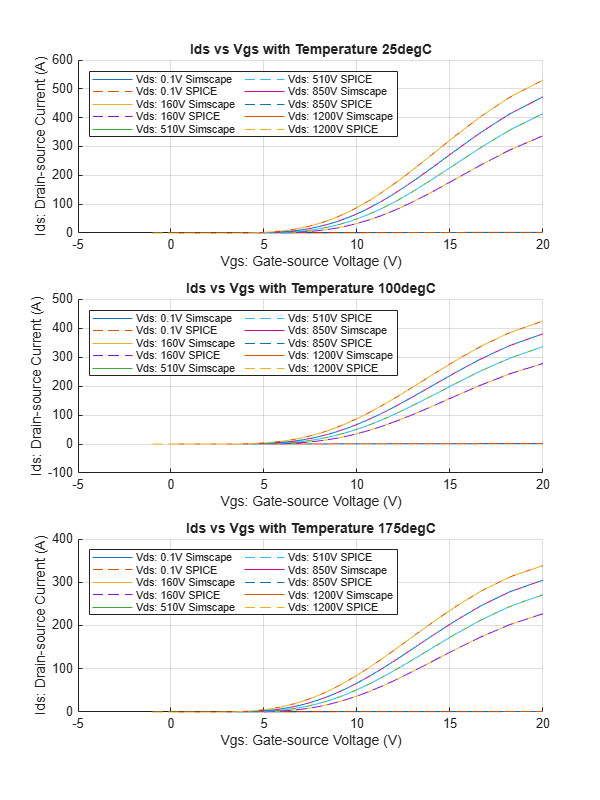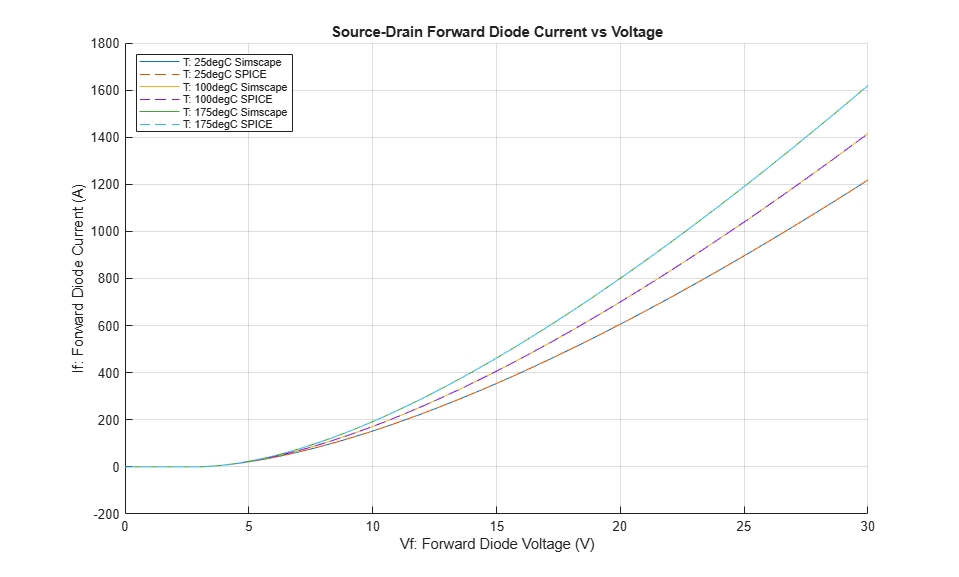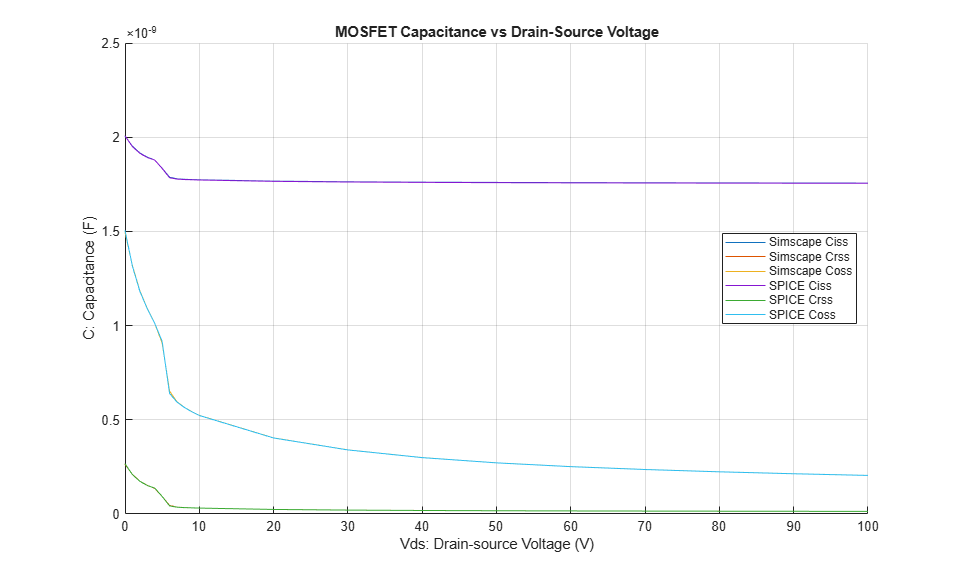# SiC MOSFET Parameterization Using Simulation Results from SPICE

This example shows how to generate lookup table data for a silicon carbide (SiC) metal-oxide-semiconductor field-effect transistor (MOSFET) from SPICE subcircuits by using the `ee.spice.semiconductorSubcircuit2lookup` function and parameterize an N-Channel MOSFET block in Simscape™ Electrical™.

### Open the SiC MOSFET Subcircuit

The subcircuit contains the SPICE model of the Infineon 1200V SiC Power MOSFET IMBG120R045M1. To open the subcircuit, at the MATLAB® command prompt, enter: edit Infineon-IMBG120R030M1.lib. You can download the SPICE library that contains the required model from the manufacturer website.

### Set the Required Lookup Table Parameters for the Function Input Arguments

To run the `ee.spice.semiconductorSubcircuit2lookup` function, you need to characterize the device by specifying the corresponding subcircuit file path, the subcircuit name, and the name-value argument pair. For more information, see the `ee.spice.semiconductorSubcircuit2lookup` documentation page.

### Generate Lookup Table Data From SPICE Subcircuit File

Use the `ee.spice.semiconductorSubcircuit2lookup` function to generate the SiC MOSFET channel, diode, and capacitance characteristics.

### Open the N-Channel MOSFET (Lookup Table-Based) Model

Open the model `SiCMOSFETFromSPICEResults.slx`### Set Lookup Table Parameters for the N-Channel MOSFET (Lookup Table-Based) Model

You can parameterize an N-Channel MOSFET (Lookup Table-Based) in your model using the generated lookup table data.

### Comparison of Characteristics

These plots compare the transfer characteristics, the diode characteristics, and the capacitance characteristics of the N-Channel MOSFET (Lookup table-based) with the SPICE subcircuit simulation results.The N-Channel MOSFET (Lookup Table-Based) model validates the power MOSFET IMBG120R045M1 against SPICE subcircuit results.